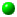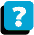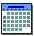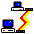Module 4.2 Notes "Comparing Two Related Samples of Numerical Data"

 Index to Module 4 Notes4.1 Comparing 2 Independent Samples of Numerical Data4.2 Comparing 2 Related Samples of Numerical Data4.3 Comparing 2 Samples Using a Nonparametric Test4.4 Comparing Multiple Samples: One Factor4.5 Comparing Multiple Samples: Two Factors4.6 Comparing Multiple Samples: A Nonparametric Test

In Module Notes 4.1, we compared two independent samples of numerical data to determine if, by inference, the means of the two populations from which the samples were drawn are equal are not. This situation requires that observations in one sample are independent from observations in the other sample. To achieve independence, the observations are randomly drawn and assigned to Sample 1 or Sample 2 in an unbiased manner (such as by flipping a coin or using a random number generator).

This module examines the interesting situation in which observations in Sample 1 are related to Sample 2. For our brand of gas car example, a possible scenario for related samples would be if we ran car number 1 with Brand of Gas A and recorded mpg. We then flushed the tank and lines, and ran the same car with Brand of Gas B and recorded mpg.

This experimental design controls for variability within each group. That is, we may have great variability in two independent samples because we chose BOCs (big overpriced cars) and CPCs (cheap plastic cars) and mixed them up and put them both in Group A and Group B. The mpg variability might be so big within the groups that we might not be able to detect any variability between the groups. By matching the cars between groups, however, we could control the variability (Cadillac XYZ is run in Group A, then the same Cadillac is run in Group B; Neon LMN is run in Group A, then run in Group B; and so forth).

You are probably aware of a lot of tests like this. A student might be given a before taking a class exam of knowledge, and then the same student is given an after taking the class exam of knowledge. The professor would hope that the means would be different!

t-Test for Mean for Paired or Matched Samples
Lets return to the car example. Worksheet 4.2.1 shows the mpg performance for seven cars assigned to receive Brand A gas. Then the same seven cars are run with Brand B gas (after purging the tanks and lines). MPG is recorded for each car. This test involves paired or matched samples since the first observation in the first group is somehow paired or matched with the first observation in the second group. In our scenario, it is the exact same car.

Worksheet 4.2.1
 Brand A Brand B 20 20 20 20.5 19 18.5 16 20 15 19 17 19 14 18

Now we want to determine if the average mpg performance with Brand A is the same as that with Brand B. The hypotheses are:

H0: Mean A = Mean B
Ha: Mean A =/= Mean B

To do this test, we use another t-Test Data Analysis Add In. Select Tools on the Standard Toolbar in Excel, Data Analysis in the pulldown menu, then t-Test: Paired Two Sample for Means. The dialog box is identical to the dialog box for the t-Tests we did in Module 4.1 Notes. My results are shown in Worksheet 4.2.2.

Worksheet 4.2.2

 t-Test: Paired Two Sample for Means Brand A Brand B Mean 17.3 19.3 Variance 5.9 0.8 Observations 7 7 Pearson Correlation 0.6 Hypothesized Mean Difference 0 df 6 t Stat -2.6 P(T<=t) one-tail 0.0198 t Critical one-tail 1.9 P(T<=t) two-tail 0.0397 t Critical two-tail 2.4

Since the p-value (two-tail; 0.0397) is less than our threshold value of 0.05, we reject the null hypothesis and claim that there is a significant difference between the means. In this case, Brand of Gas B is outperforming Brand of Gas A.

Wait a minute!! When I ran the identical data with a t-Test for Independent Samples in Module Notes 4.1 we got a p-value of 0.076 and did not reject the null hypothesis - the means were equal (any difference was due to chance and not significant). This is a great lesson. We can use statistical test to prove a point - but it might be the wrong test and it was unethical for us to use it.

In Module 4.1, when we treated the samples as independent, it doesn't matter how we enter the data for each sample since the data are independent. But when we have the situation that the data are related between the two samples (Module 4.2), we are required to enter the pairs in the same row. This allows the statistical analysis to factor out any variability between rows (also known as within group variability) and have a controlled comparison between columns (also known as between group variability).

This is a powerful test procedure that has many applications when there are paired observations. This wraps up Module 4.2., although I believe I owe you one more note.

In our tests of means for two independent or paired sample groups, I always used the two-tailed p-value results because the alternative hypotheses were non-directional two-tailed. If an alternative hypothesis of interest to you is Mean A < Mean B or Mean A > Mean B, then you would need to use the one-tail p-value result.

References:

Anderson, D., Sweeney, D., & Williams, T. (2001). Contemporary Business Statistics with Microsoft Excel. Cincinnati, OH: South-Western, Chapter 10 (Section 10.3).About the CourseModule ScheduleWebBoard